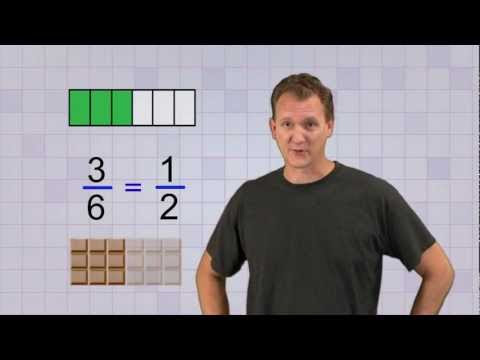## Posts

### email

Hello Grade 7's please start using your email and password for google classroom

### Simplifying FractionsPlease watch short video on simplifying fractions: Here is another example: So, the steps are: 1. What are the factors for the numerator and denominator? 2. Are there any numbers that can be cancelled out? 3. Are there any composite numbers, if so choose only prime numbers if possible. Your answer should be simplified, meaning you can't make the fraction smaller. Do the following assignment: Loading…

### Factors January 29,2021Please watch video on factors: Factors: What are the factors of 12? To solve this , you ask yourself what numbers can I use to multiply to equal 12?  2x6= 12, so 2, 6 are factors of 12. 3x4=12, 3,4 are factors of 12. 1x12=12, so 1, 12 are factors of 12. So, all the numbers 1,2,3,4,6,12 are all factors of 12. The key is, when you are asked to find the factors of 12, you ask yourself what numbers can I multiply with to get 12. Loading…

### Math: Equivalent Fractions: Using Equivalency Blocks- Jan.26,2010Here is another way to show equivalency using cubes: If you look at 1/2, is 1/2 equivalent to 1/3? No, it isn't. If you look at 1/2 and 1/3, the way to check if they are equivalent using the cubes is to see if there's a line that goes through 1/2 and 1/4: How many 1/4's do you need to make 1/2? 2/4 is the answer. You can see that there is a line that goes straight through 1/2 and 2/4. You can also multiply the numerator and denominator of 1/2 =2/4.  So, to get 2/4, you multiply 2 with the numerator and then denominator of 1/2.  Or you can do this: Now it's time to do some equivalent fractions. Loading…

### Math: Equivalent Fractions: January 25,2021January 25,2021 Watch the video on Equivalent Fractions, then follow by doing worksheet. Rule to find equivalent fractions: Equivalent fractions can only be made by multiplying or dividing and Whatever you do the numerator (top #), you do to the denominator ( bottom #). You can use any number you want as long as you multiply the numerator and denominator with the same number. Loading…

### Representing fractions using setsWatch the video on representing fractions, then do following assignment. Loading…

### First They Came

Follow along as I read the poem:  Loading…